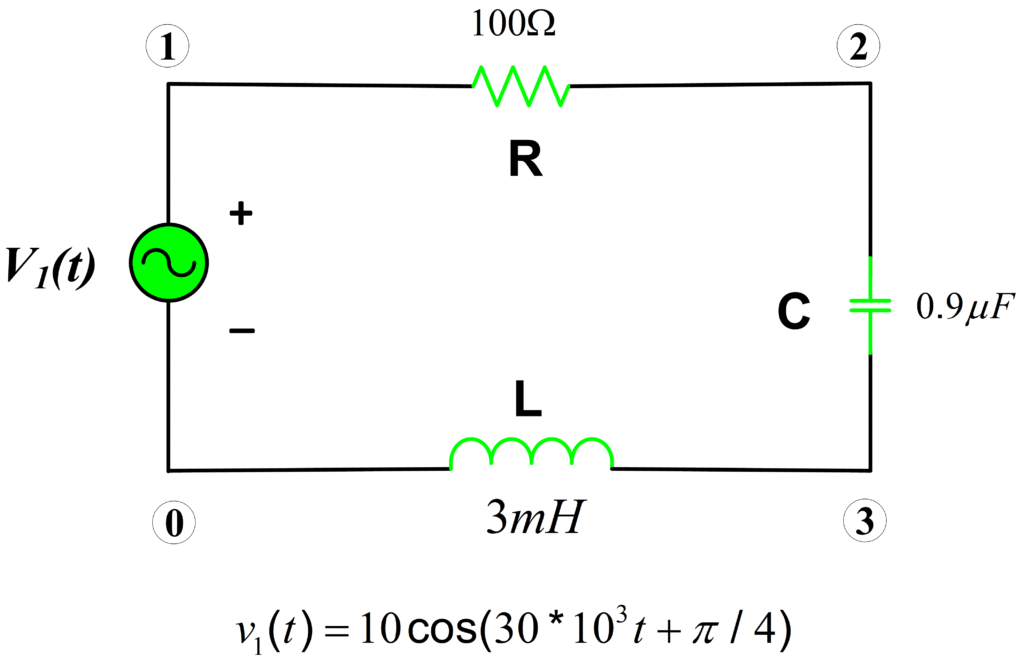Home / Circuits with Matlab / RLC Circuit Transfer Function Calculation using Matlab

# RLC Circuit Transfer Function Calculation using Matlab

Want create site? Find Free WordPress Themes and plugins.

Plot the magnitude and the phase response of the voltage transfer function of series RLC circuit for frequencies from 10 Hz to 100kHz.:Here, we will compute the phase and the magnitude of the voltage transfer function Vo/V1 for frequencies ranging from 10 Hz to 100 kHz. The transfer function can be determined by the following relation:

$H(f)=\frac{{{V}_{o}}}{{{V}_{1}}}=\frac{{{Z}_{C}}}{{{Z}_{C}}+{{Z}_{L}}+R}$

A transfer function is simply a ratio between input and output.

Whereas:

\begin{align} & {{Z}_{C}}=\frac{1}{j\omega C} \\ & {{Z}_{L}}=j\omega L \\\end{align}

Now, let’s compute the transfer function using Matlab:

## Matlab Code RLC Circuit Transfer Function

%Transfer Function Calculation for an AC Circuit
clear all;close all;clc
%% Circuit Parameters
R= 30; % Resistance (30 Ohm)
L= 0.7e-3; % Inductance (0.7 mH)
C= 1.5e-6; % Capacitance (1.5 microfarad)
% Please see "help logspace" in order to understand how does logspace work?
f= logspace(1,5); % Frequency range between 10 Hz and 100 kHz
omega= 2*pi.*f; % Angular Frequency
ZC= 1./(j.*omega.*C); % Capacitive Reactance
ZL= j.*omega.*L; % Inductive Reactance
Hf=ZC./(ZC+ZL+R); % Transfer Function (V0/V1)
%% Plot the phase and the magnitude response of a transfer function
%Magnitude Plot
subplot(211)
%loglog(...) is the same as PLOT(...), except logarithmic scales are used for both the X- and Y- axes.
loglog(f,abs(Hf))
title('Magnitude')
xlabel('Frequency (Hz)')
ylabel('Amplitude')
% Please see "help axis" in order to understand how does axis work.
axis([10 1e5 1e-3 10]) % Manual axis adjustment

%Phase Plot
subplot(212)
%semilogx(...) is the same as PLOT(...), except a logarithmic (base 10) scale is used for the X-axis.
semilogx(f,angle(Hf))
title('Phase')
xlabel('Frequency (Hz)')
axis([10 1e5 -3.5 0.5]) % Manual axis adjustment
%==============================================


RLC Circuit Transfer Function Frequency Response: